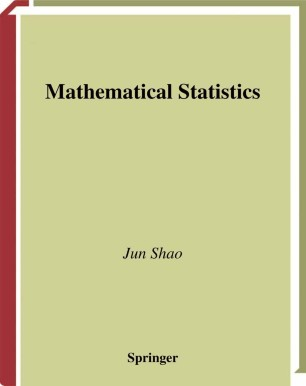# Mathematical Statistics

• Jun ShaoTextbook

Part of the Springer Texts in Statistics book series (STS)

1. Front Matter
Pages i-xiv
2. Pages 1-60
3. Pages 61-126
4. Pages 127-192
5. Pages 193-276
6. Pages 277-344
7. Pages 345-420
8. Pages 421-488
9. Back Matter
Pages 489-530

### Introduction

This book is intended for a course entitled Mathematical Statistics o?ered at the Department of Statistics, University of Wisconsin-Madison. This course, taught in a mathematically rigorous fashion, covers essential - terials in statistical theory that a ?rst or second year graduate student typically needs to learn as preparation for work on a Ph. D. degree in stat- tics. The course is designed for two 15-week semesters, with three lecture hours and two discussion hours in each week. Students in this course are assumed to have a good knowledge of advanced calculus. A course in real analysis or measure theory prior to this course is often recommended. Chapter 1 provides a quick overview of important concepts and results in measure-theoretic probability theory that are used as tools in the rest of the book. Chapter 2 introduces some fundamental concepts in statistics, including statistical models, the principle of su?ciency in data reduction, and two statistical approaches adopted throughout the book: statistical decision theory and statistical inference. Each of Chapters 3 through 7 provides a detailed study of an important topic in statistical decision t- ory and inference; Chapter 3 introduces the theory of unbiased estimation; Chapter 4 studies theory and methods in point estimation under param- ric models; Chapter 5 covers point estimation in nonparametric settings; Chapter 6 focuses on hypothesis testing; and Chapter 7 discusses int- val estimation and con?dence sets.

### Keywords

Likelihood mathematical statistics probability probability theory statistical theory statistics

• Jun Shao
• 1